Date: Fri, 28 Aug 1998 21:34:26 -0700
From: Lorraine
Hi,

Consider the region in the first quadrant bounded by the x and y axes, the vertical line x=3 and the curve y = 1 / (x squared + 3). Determine the volume of the solid by rotating this region about the x-axis. Now that is the first part.
I then have to find the coordinates of the centroid of the solid by rotating this region about the x-axis.

Thanks.
Lorraine

Hi Lorraine,
I used the disk method to find the volume. A disk of thickness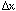and height y, rotated about the x-axis gives a volume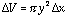. Since y = 1/(x squared + 3), the volume required is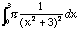.

The exprression (x^2+3) should make you think of a tangent substitution, so let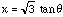then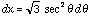, and the integrand becomes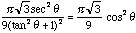.

Integrating the cosine squared is accomplished by using the identity that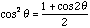.
After some algebra and arithmetic I got the volume to be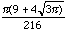.

I hope this helps,
Harley

Go to Math Central